# [Algorithms I] Week 6 Hash Tables

Can we do better than BST if we do not need ordered operations ?

(No compare methods, use equals method)

Idea: save items in an array.
Hash function: method for calclulating the array index of a key.

Issues:

• computing hash function
• equality tests
• collision resolution

# 1. Hash Functions

Goal: scramble the keys uniformly to produce a table index.

• effcient to compute
• all indices are equally likely for any key

challenge: need different approach for different key types.

## Java's hashing

All java object has a methode `int hashCode()`
requirement:

• if x.equals(y) ⇒ x.hashCode()==y.hashCode()
• (hopefully) if x.equals(y)==false ⇒ x.hashCode!=y.hashCode()

• Default implementation: memory address for x.

• Custom implementations for standard types: Integer, Double, String, File, URL, Date...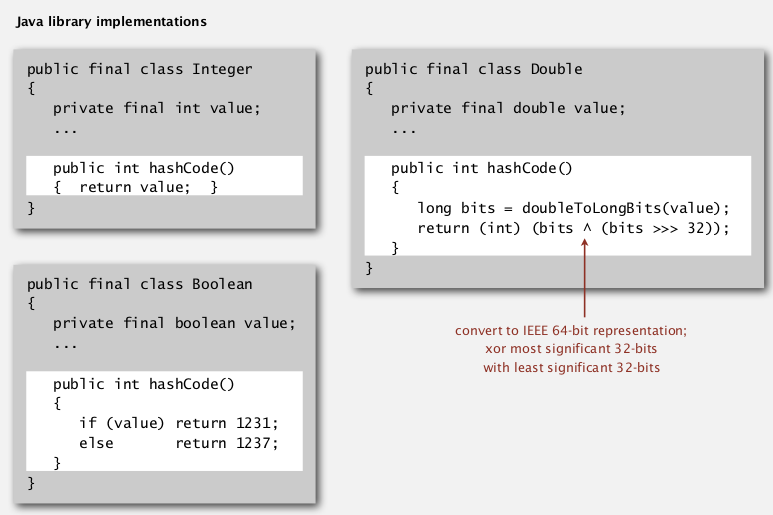Strings: Horner's method — compute a polynome.recipe for user-defined types:## Modeular hasing

(hash code VS hash function)

• Hash code: 32-bit integer between -2^31 and 2^31-1 (can be negative!!)
• Hash function: integer between 0 and M-1 (used directly as array index, should >=0)

BUG code:

```private int hash(Key k){
return k.hashCode()%M;
}
```

→ bug: number returned can be negative !

1-in-billion bug code:
`return Math.abs(k.hashCode()) % M;`

→ bug: Math.abs() returns negative numbers for -2^31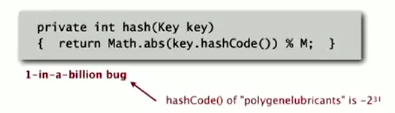correct code:
just take the first 31 bits: x&0x7fffffff
`return k.hashCode()&0x7fffffff % M;`

## uniform hasing assumption

each key is equally likely to hash to an integer between 0 and M-1# 2. Separate Chaining

one strategy for collision resolution.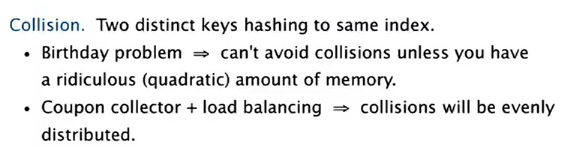idea: Using a table of size M < N, build a list for each of the table positions.## implementation

```public class SeparateChainingHashST<Key, Value>{
private int M = 97;
private Node[] st = new Node;
private static class Node{
Object key, val; // no generic array creation!
Node next;
public Node(Key k, Value v, Node nxt){...}
}
int hash(Key k){
return (k.hashCode()&0x7fffffff) % M;
}
public Value get(Key k){
int h = hash(k);
for(Node x=st[h]; x!=null; x=x.next)
if(k.equals(x.val)) return (Value)x.val; //cast
return null;
}
public void put(Key k, Value v){
int h = hash(k);
for(Node x=st[h]; x!=null; x=x.next)
if(k.equals(x.val))
{x.val = v; return;}
st[h] = new Node(k,v,st[h]);
}
}
```

## analysis

proposition
Under the assumption of uniform hashing, the number of keys in a list is within a constant factor to N/M.
proof.
binominal distribution.

M times faster than sequential search
→ typical choice: M ~ N/5

# 3. Linear Probing

Another approch for collision resolution.

Use an array of size M>N, when a key collides, find next open slot.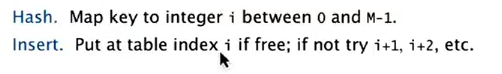• insert: when occupied, move pointer until got open slot
• search: when not found, move pointer until find or reach open slot
• delete: set key to null, then for all items behind this key: set to null, then insert this key......

array M must be larger than N ⇒ array resizing is necessary.

## implementation

```public class LinearProbingHashST<Key, Value>{
private int M, N;
private Object[] keys;//cannot use generic array
private Object[] vals;
public void put(Key k, Value v){
int i = hash(k);
for(;keys[i]!=null;i=(i+1)%M)
if(keys[i].equals(k))
{vals[i]=v; return;}
keys[i]=k;
vals[i]=v;
}
public Value get(Key k){
int h=hash(k);
for(;keys[h]!=null;h=(h+1)%M)
if(k.equals(keys[h])) return (Value)vals[h];//ugly cast
return null;
}
}
```

## cluster

def. cluster
A contiguous block of items.

New keys are more likely to hash into a cluster.

Knuth parking pb: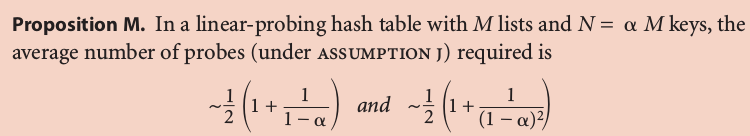(当年Knuth就是在证明了这个以后决定写那套书)

Typical choice: N/M ~ 1/2 (3/2 for search hit and 5/2 for search miss)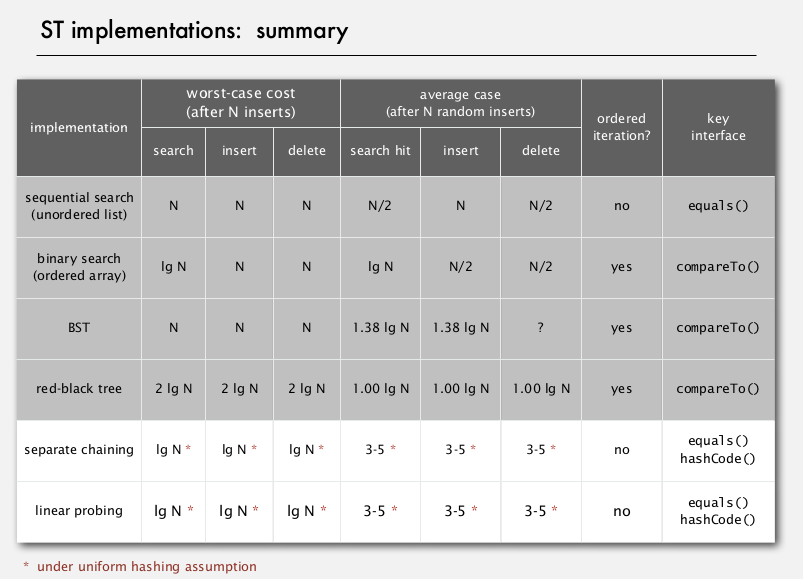# 4. Hash Table Context

widely used in applicataions.

cost for computing hash VS cost for searching:
hashCode() for strings in java 1.1: exemain only 8-9 evenly spaced characters to save time.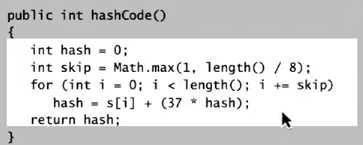uniform hashing assumption: performance not guaranteed...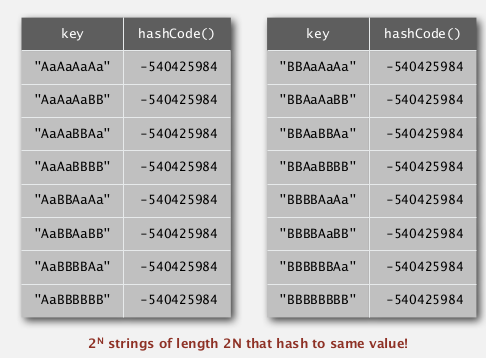one-way hash functions
Hard to find a key that hash to a desired value, or 2 keys having the same hash value.used for fingurprints or store passwords, but too expensive for ST implementations.

## Seperate chaining VS linear probing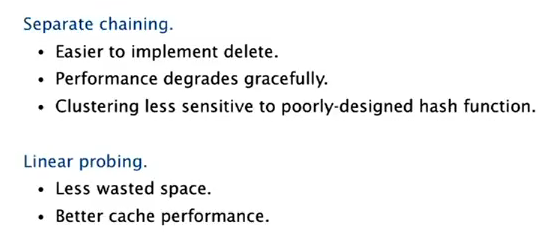## variations## ST choice: hash tables VS balanced search trees